Graphing Rational Functions Terms of Use    Contact Person: Donna RobertsDirections: The following questions pertain to graphs of rational functions.
Do not use your graphing calculator, unless instructed to do so.

1.
At what x-value location(s) would you find vertical asymptotes for the function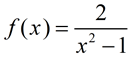?
Choose:
 x = 1 only x = -1 only x = ± 1 x = 2

2.
Given the function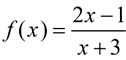:
a) What is the x-intercept of this graph?
Choose:
 (½, 0) (- ½, 0) (3, 0) (-3, 0)

b)
What is the equation of the vertical asymptote?

Choose:
 x = ½ x = -3 y = ½ y = -3

3.
Given the graph shown at the right in a 10 x10 viewing window. Which of the following equations could have possibly been used to produce this graph?
(Do not use your graphing calculator!)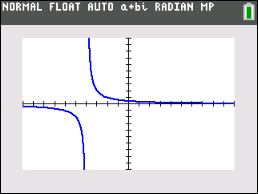Choose: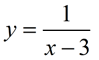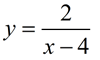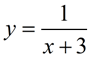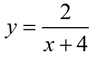4.
Which of the following functions has x = 0 as a vertical asymptote?
(Do not use your graphing calculator!)

Choose:
 y =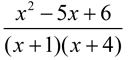y =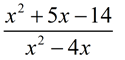y =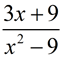y =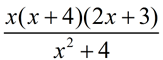5.
The characteristics of the following function are that its zeros are at x = -1 and x = 3 and its vertical asymptote is at x = 0.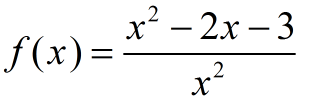Choose:
 Yes No

6.
Which of these functions has no vertical asymptotes?
(Check this one out on your graphing calculator.)
Choose: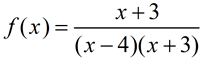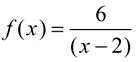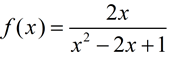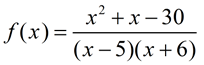7.
Given the graph of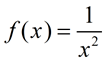shown at the right. Which of the following statements describes the behavior of the graph as x approaches positive infinity?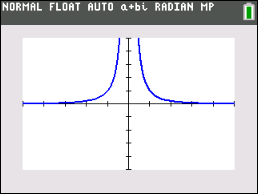Choose:
 f (x) approaches positive infinity f (x) approaches one f (x) approaches negative infinity f (x) approaches zero

8.
Find the x-intercept for the function: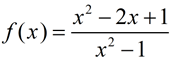(Do not use your graphing calculator!)Choose:
 (0,1) (1,0) (-1,0) none